# Exercises: Chapter 4, Section 3

1. Let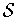be the set of all singular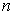-cubes, and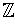the integers. An-chain is a function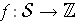such that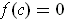for all but finitely many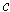. Define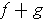and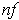by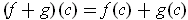and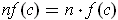. Show thatandare-chains if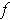and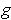are. If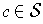, letalso denote the functionsuch that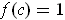and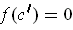for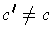. Show that every-chaincan be written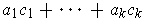for some integers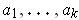and singular-cubes.

Since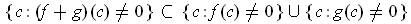and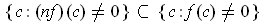, the functionsandare-chains ifandare.

The second assertion is obvious since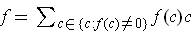.

2. For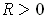andan integer, define the singular 1-cube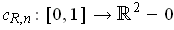by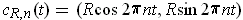. Show that there is a singular 2-cube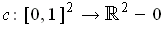such that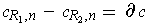.

Defineby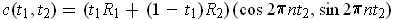where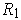and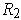are positive real numbers. The boundary ofis easily seen to be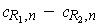.

3. Ifis a singular 1-cube in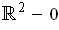with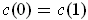, show that there is an integersuch that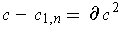for some 2-chain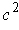.

Given, let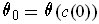where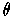is the function of Problem 3-41 extended so that it is 0 on the positive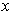-axis. Let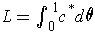so that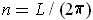is an integer because. Define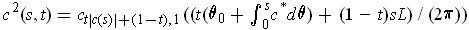. One has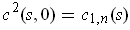and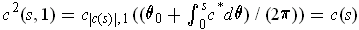. On the other boundaries,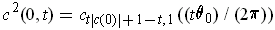and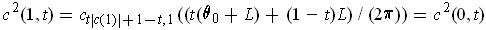. So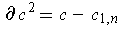, as desired.